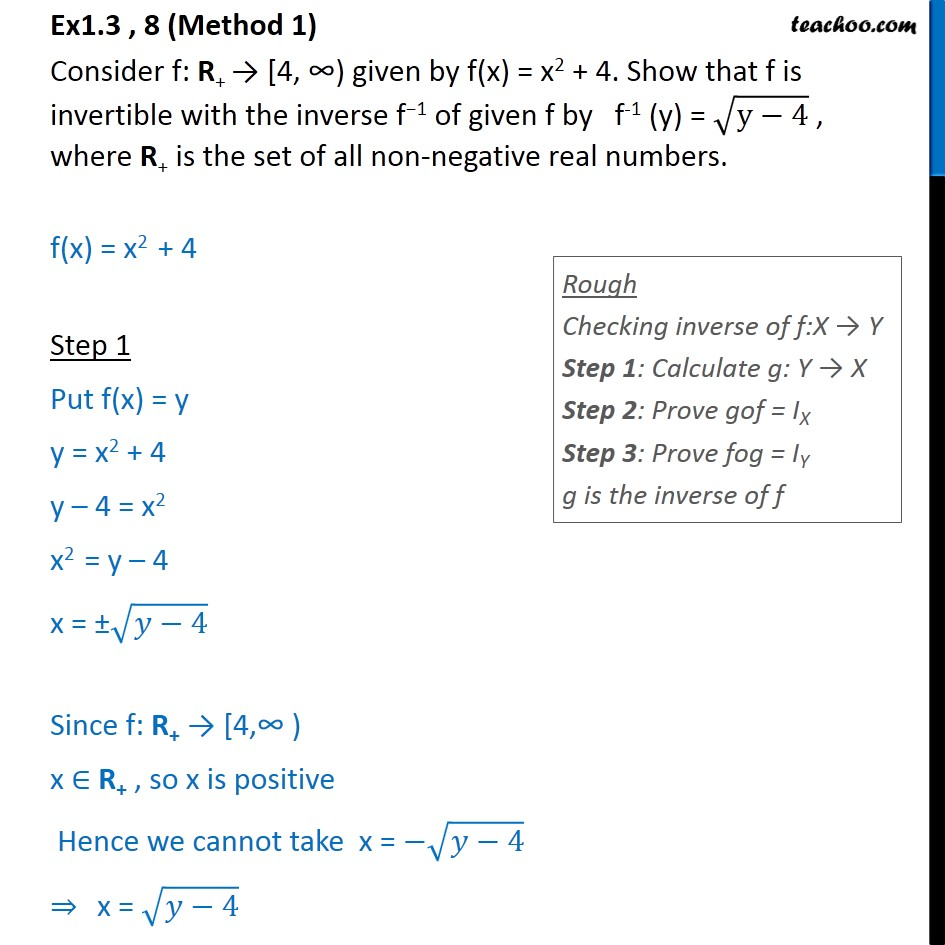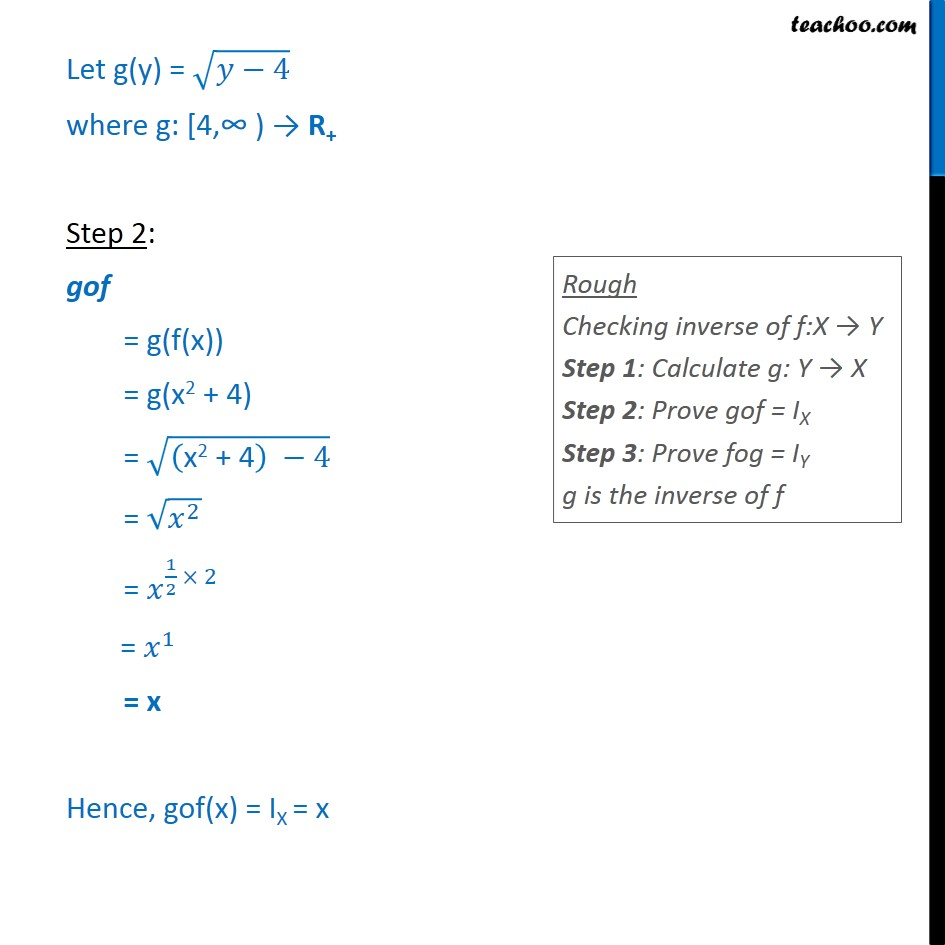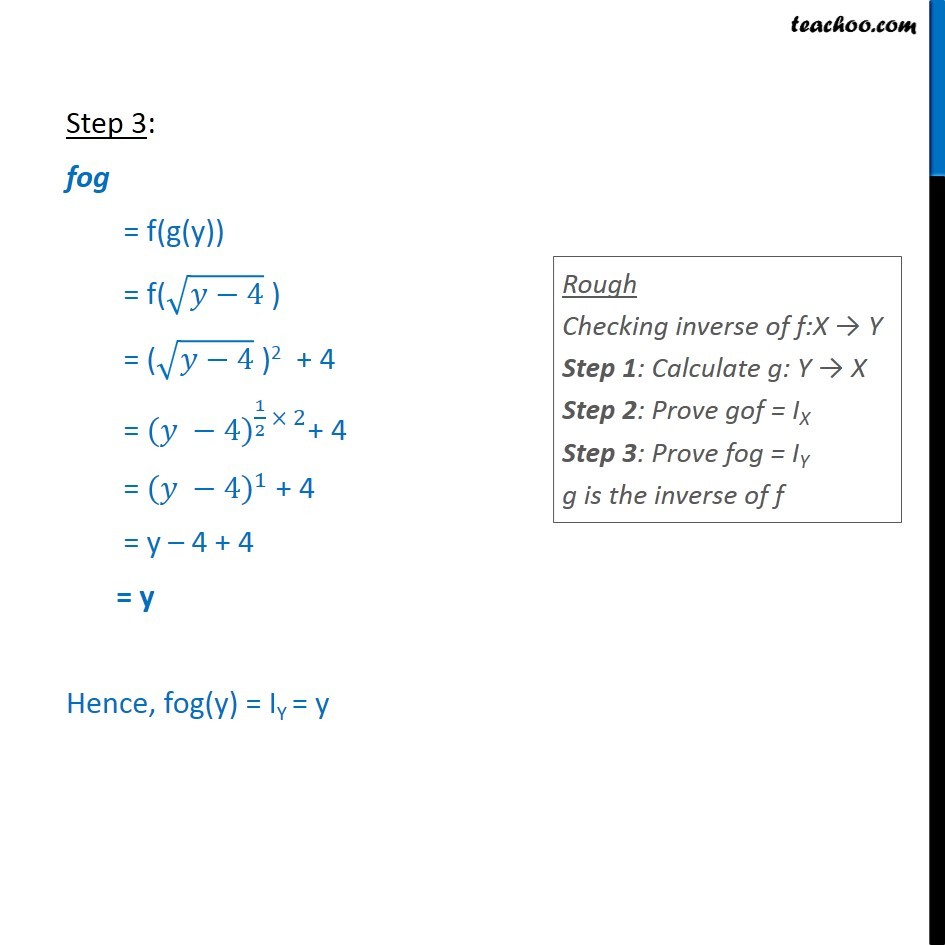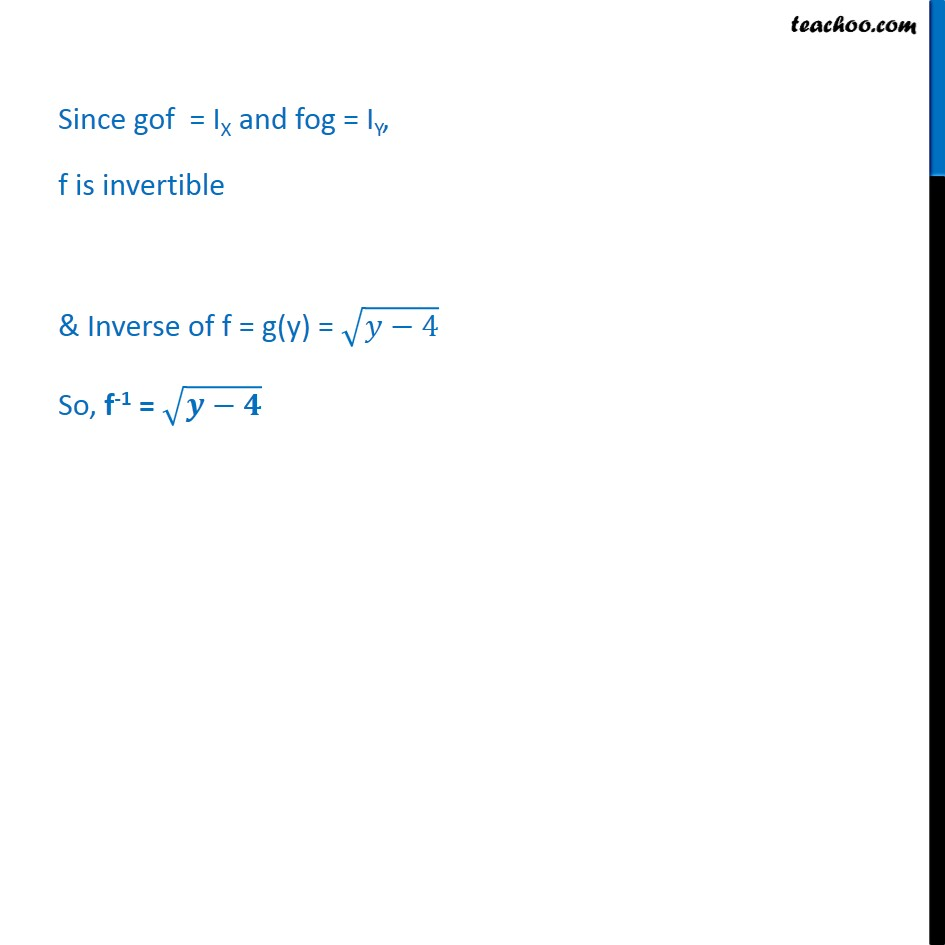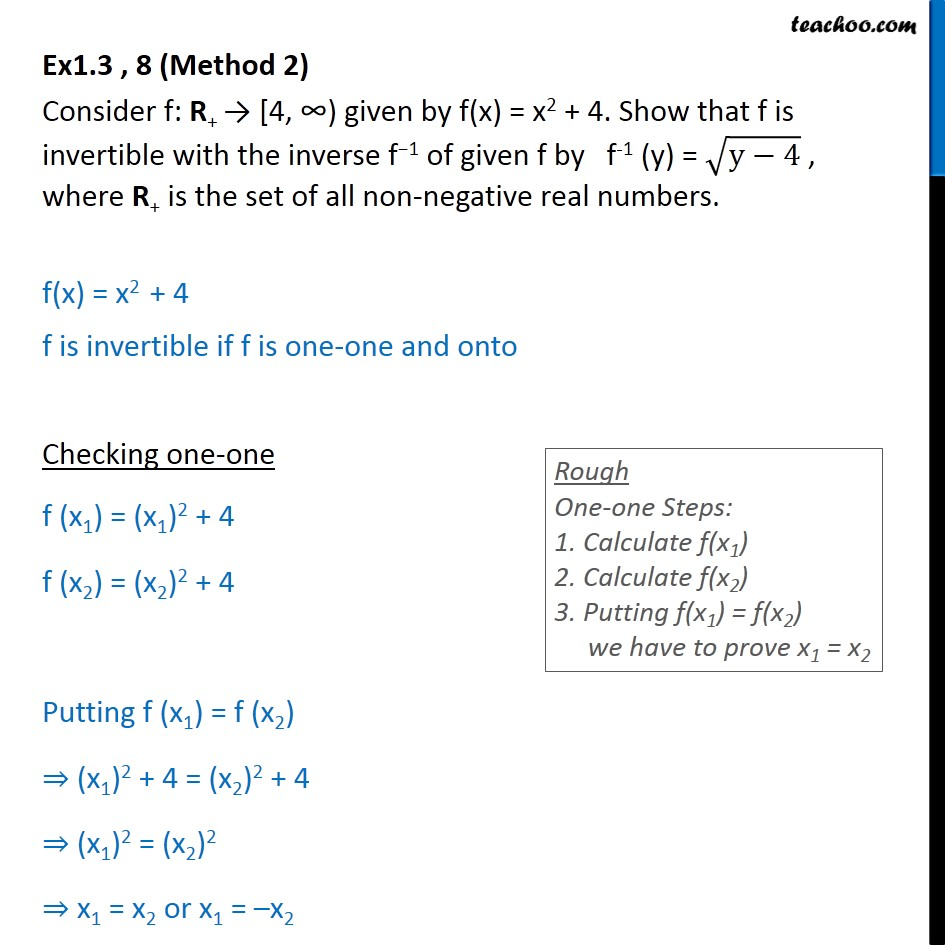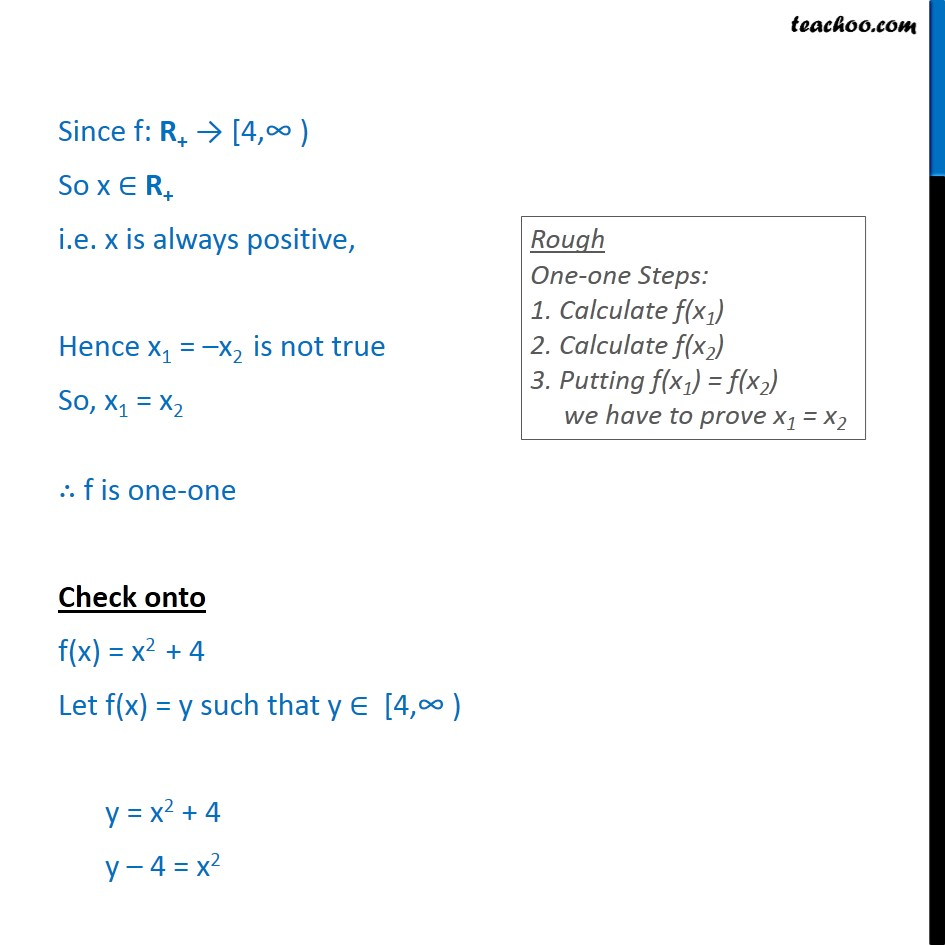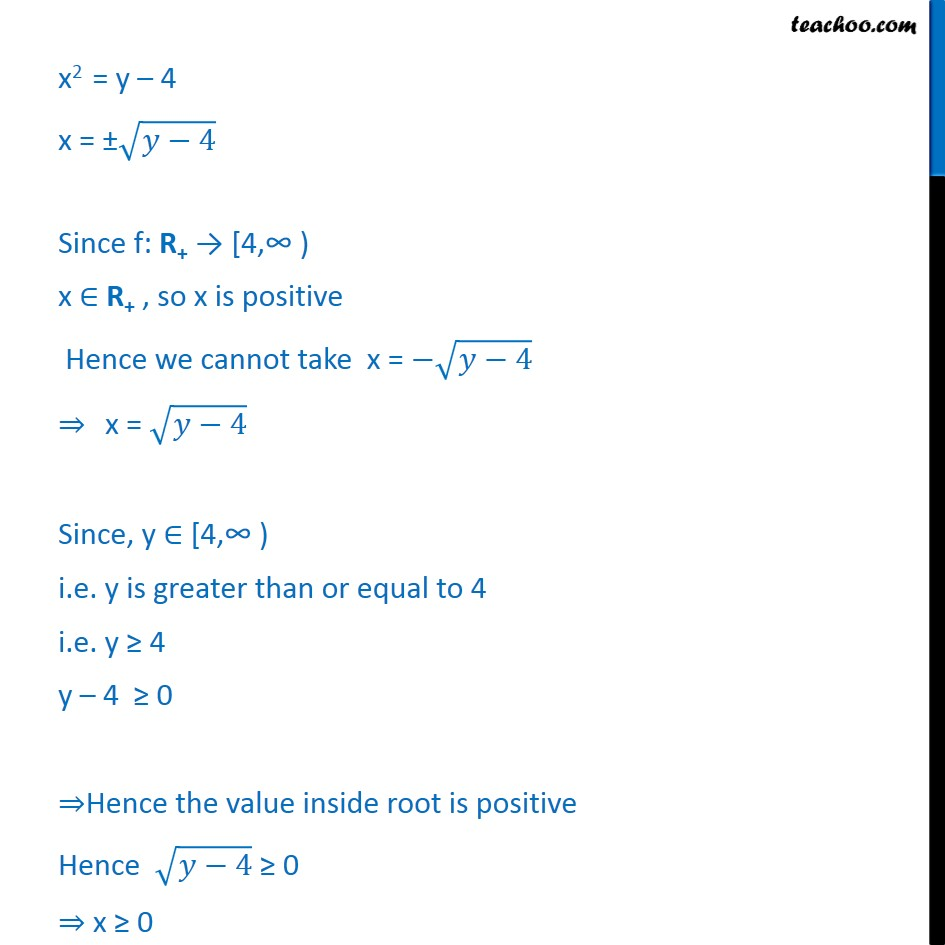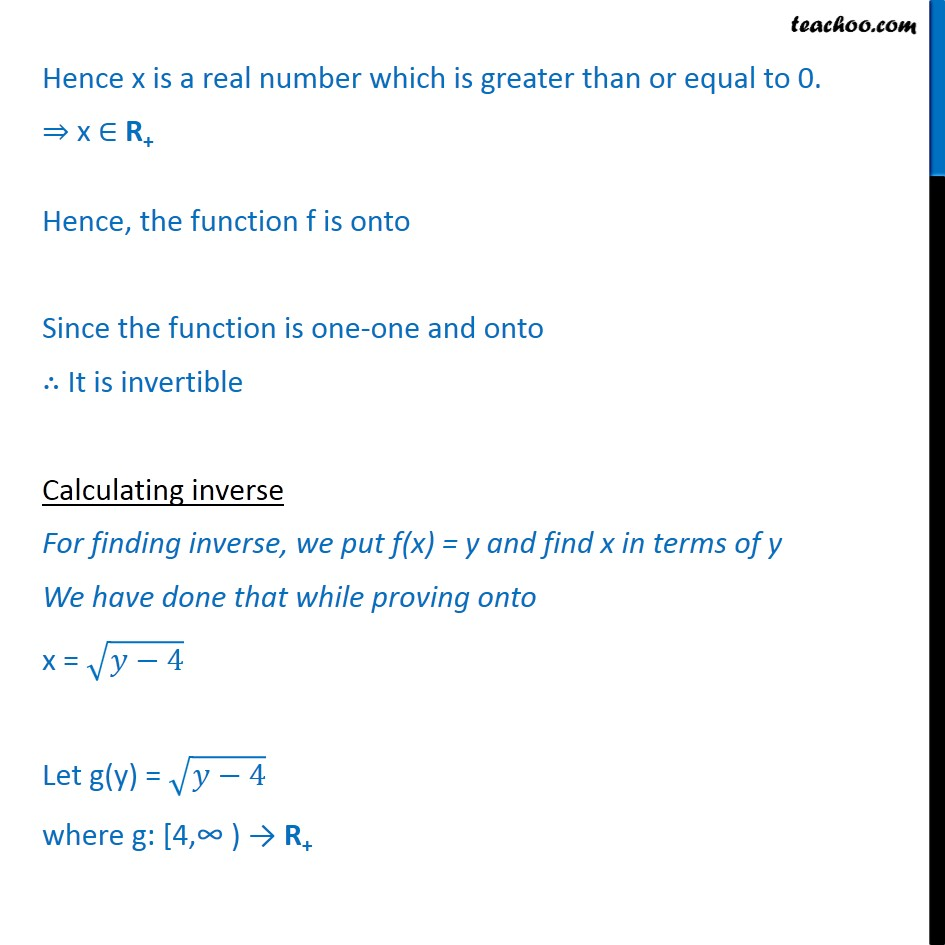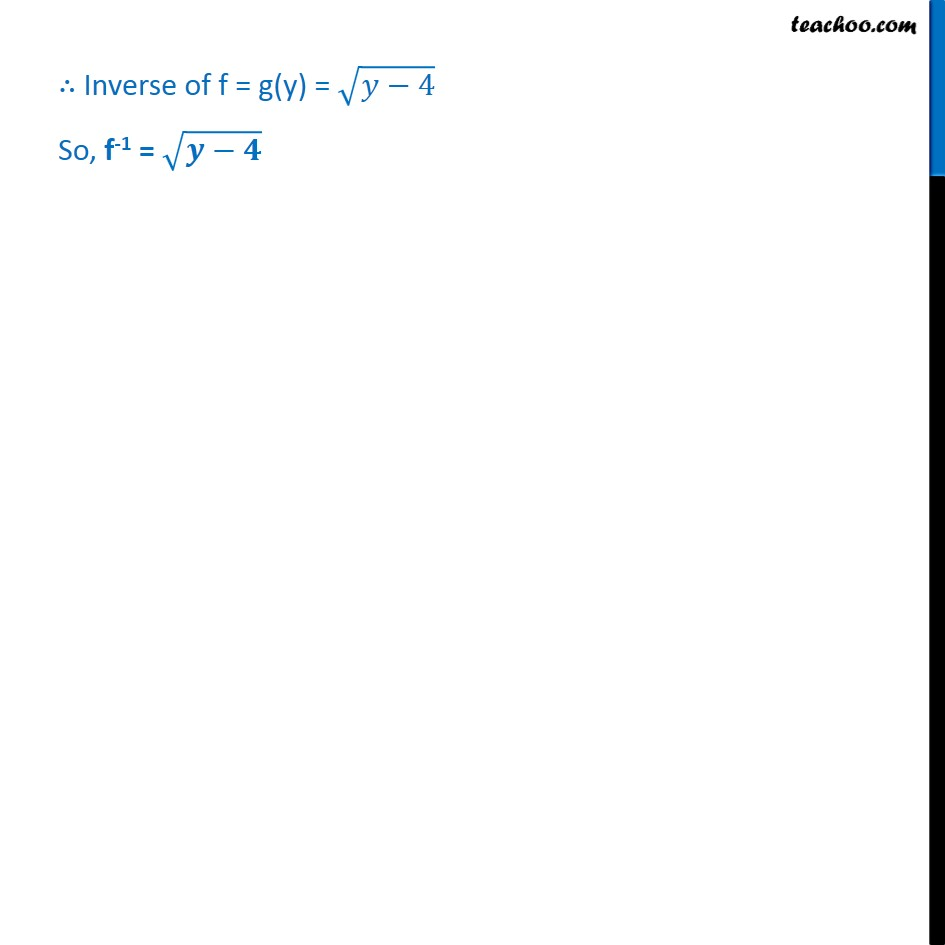1. Chapter 1 Class 12 Relation and Functions
2. Serial order wise
3. Ex 1.3

Transcript

Ex1.3 , 8 (Method 1) Consider f: R+ [4, given by f(x) = x2 + 4. Show that f is invertible with the inverse f 1 of given f by f-1 (y) = y 4 , where R+ is the set of all non-negative real numbers. f(x) = x2 + 4 Step 1 Put f(x) = y y = x2 + 4 y 4 = x2 x2 = y 4 x = 4 Since f: R+ [4, ) x R+ , so x is positive Hence we cannot take x = 4 x = 4 Let g(y) = 4 where g: [4, R+ Step 2: gof = g(f(x)) = g(x2 + 4) = x2 + 4 4 = 2 = 1 2 2 = 1 = x Hence, gof(x) = IX = x Step 3: fog = f(g(y)) = f( 4 ) = ( 4 )2 + 4 = ( 4) 1 2 2 + 4 = ( 4) 1 + 4 = y 4 + 4 = y Hence, fog(y) = IY = y Since gof = IX and fog = IY, f is invertible & Inverse of f = g(y) = 4 So, f-1 = Ex1.3 , 8 (Method 2) Consider f: R+ [4, given by f(x) = x2 + 4. Show that f is invertible with the inverse f 1 of given f by f-1 (y) = y 4 , where R+ is the set of all non-negative real numbers. f(x) = x2 + 4 f is invertible if f is one-one and onto Checking one-one f (x1) = (x1)2 + 4 f (x2) = (x2)2 + 4 Putting f (x1) = f (x2) (x1)2 + 4 = (x2)2 + 4 (x1)2 = (x2)2 x1 = x2 or x1 = x2 Since f: R+ [4, So x R+ i.e. x is always positive, Hence x1 = x2 is not true So, x1 = x2 f is one-one Check onto f(x) = x2 + 4 Let f(x) = y such that y [4, y = x2 + 4 y 4 = x2 x2 = y 4 x = 4 Since f: R+ [4, x R+ , so x is positive Hence we cannot take x = 4 x = 4 Since, y [4, i.e. y is greater than or equal to 4 i.e. y 4 y 4 0 Hence the value inside root is positive Hence 4 0 x 0 Hence x is a real number which is greater than or equal to 0. x R+ Hence, the function f is onto Since the function is one-one and onto It is invertible Calculating inverse For finding inverse, we put f(x) = y and find x in terms of y We have done that while proving onto x = 4 Let g(y) = 4 where g: [4, R+ Inverse of f = g(y) = 4 So, f-1 =

Ex 1.3